# College Chemistry : Atoms and Elements

## Example Questions

### Example Question #6 : Periodic Trends

Which atom is the most electronegative?

Phosphorus

Sulfur

Chlorine

Aluminium

Silicon

Chlorine

Explanation:

Electronegativity refers to the ability of an atom to attract and bind electrons.  If the valence electrons are less then half full, then it takes less energy to lose an electron than gain electron making these atoms less electronegative. If the valence electrons are more than half full, then it takes less energy to gain electrons compared to losing an electron; therefore these electrons are more electronegative. The periodic table can be used to predict the electronegativity of the atom and how it compares to other atoms. As you move left to right of the periodic table, the electronegativity increases because the atoms on the right side of the periodic table have more valence electrons, and smaller radii. As you move down the periodic table the electronegativity decreases because the atomic number increases resulting in a greater distance between valence electrons and the nucleus causing less pull on the valence electrons.

Using the electronegativity periodic trend, we can predict which of the atom's is most electronegative. Aluminium, silicon, phosphorous, sulfur, and chlorine are all in the same row in the periodic table. Therefore, chlorine is the most electronegative because it is the farthest right on the periodic table so it has the most valence electrons and it will cost less energy to gain an electron than lose an electron.

### Example Question #7 : Periodic Trends

Which of the following atoms in the largest?

Aluminium

Phosphorous

Sulfur

Sodium

Chlorine

Sodium

Explanation:

The atomic radii is the size of an atom when it is not bonded to any other atoms. The periodic table can be used to estimate the size of the atomic radii of atoms. As you move down the periodic table the atomic radii increase, but as you move from left to right on the periodic table, the size of the atomic radii decrease.

Sodium, aluminium, phosphorus, sulfur, and chlorine are all in the same row of the periodic table. Since the size of the atomic radii decreases as you move from left to right on the periodic table, the element furthest to the left will be the largest. Therefore, sodium is the largest atom out of the group.

### Example Question #8 : Periodic Trends

Which element is the least electronegative?

Sulfur

Selenium

Polonium

Tellurium

Oxygen

Polonium

Explanation:

Electronegativity refers to the ability of an atom to attract and bind electrons.  If the valence electrons are less then half full, then it takes less energy to lose an electron than gain electron making these atoms less electronegative. If the valence electrons are more than half full, then it takes less energy to gain electrons compared to losing an electron; therefore these electrons are more electronegative. The periodic table can be used to predict the electronegativity of the atom and how it compares to other atoms. As you move left to right of the periodic table, the electronegativity increases because the atoms on the right side of the periodic table have more valence electrons. As you move down the periodic table the electronegativity decreases because the atomic number increases resulting in a greater distance between valence electrons and the nucleus causing less pull on the valence electrons.

Using the electronegativity periodic trend, we can predict which of the atom's is least electronegative. Oxygen, sulfur, selenium, tellurium, and polonium are all in the same group of the periodic table. Therefore, polonium is the least electronegative because it is closest to the bottom of the periodic table and has the largest atomic numbers. Since polonium has the largest atomic number, there is a greater distance between the valence electrons and the nucleus resulting in a decreased pull on the valence electrons. Therefore, it is easier to lose a valence electron compared to atom's with a smaller atomic number.

### Example Question #1 : Electron Configurations

What is the electron configuration for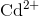?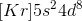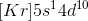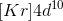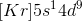Explanation:

Cadmium normally haselectrons, butonly haselectrons.

The normal electron configuration for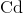is as follows: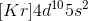Since the cadmium is losingelectrons, it must lose them from the highest energy shell. In this case, this means the cadmium will be losing its electrons in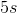Thus, the electron configuration foris.

### Example Question #2 : Electron Configurations

What is the electron configuration for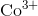?

There is no valid electron configuration for.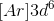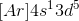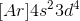Explanation:

The normal electron configuration for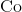is as follows: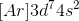Since it is losingelectrons, the element must lose the electrons from the highest energy shell first. Thus, the element loseselectrons from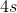andelectron for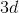.

The electron configuration foris then### Example Question #3 : Electron Configurations

What is the electron configuration for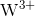?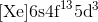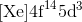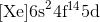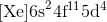Explanation:

Start by finding the noble gas core. For tungsten, this will be xenon as this is the noble gas that is closest to it.

The normal electron configuration for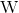is as follows: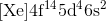Recall that electrons are lost in the highest energy level subshell first.has lostelectrons. It will lose the first two electrons from the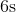shell, then it will loseelectron from the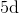shell, giving it the following electron configuration:### Example Question #4 : Electron Configurations

What is the electron configuration for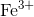?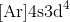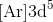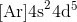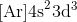Explanation:

Start by finding the noble gas core. For iron, this will be argon as this is the noble gas that is closest to it.

Next, recall that since the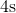orbitals are higher in energy that the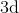orbitals, electrons will be lost from theorbital first.

The normal electron configuration for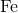is as follows: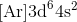has lostelectrons. It will lose the first two electrons from theshell, then it will loseelectron from theshell, giving it the following electron configuration:### Example Question #5 : Electron Configurations

When an electron moves from a lower energy state to a higher energy state, the electron __________.

neither absorbs nor releases energy

None of these. An electron cannot move from a lower energy state to a higher energy state.

both absorbs and releases energy

releases energy

absorbs energy

absorbs energy

Explanation:

Electrons of an atom are located within electronic orbitals around a nucleus. The electrons of each atoms have their own specific energy level called principal energy level. When electrons are excited by absorbing energy the electrons can jump to a high energy level. Then when an electron drops back to a lower energy level the electron emits the energy. Therefore, when an atom moves from a lower energy state to a higher energy state. the electrons absorb energy.

### Example Question #6 : Electron Configurations

What is the full electron configuration of sodium?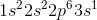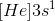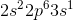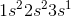Explanation:

Each element has a unique electron configuration that represents the arrangement of electrons in orbital shells and sub shells. There are four different orbitals, s, p, d, and f that each contain two electrons. The p, d, and f orbitals contain subshells that allow them to hold more electrons. The orbitals for an element can be determined using the periodic table. The s-block consists of group 1 and 2 (the alkali metals) and helium. The p-block consists of groups 3-18. The d-block consists of groups 3-12 (transition metals), and the f-block contains the lanthanides and actinides series. Using this information we can determine the full electron configuration of sodium.

To do this, start at hydrogen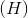located at the top left of the periodic table. Hydrogenand helium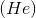are in the first s orbital and account for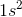. Next, we move to the second s-orbital that contains lithium (Li) and beryllium (Be), which accounts for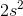. Then we move to boron, carbon, nitrogen, oxygen, fluorine, and neon, which are all in the p-block and account for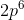. There is no 1p orbital. Finally, we are at sodium, which is in the s-block and accounts for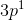. Therefore the full electron configuration of sodium is### Example Question #7 : Electron Configurations

What is the electron configuration of iodine in nobel gas notation?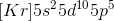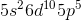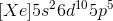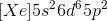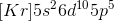The nobel gas configuration is a short hand to writing out the full electron configuration. To do this, start at the nobel gas that come before the element of interest. In the case of iodine, the nobel gas is krypton. Therefore, the electron configuration will begin with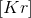, and this will be the new starting place for the electron configuration.
After krypton comes the s-block, which contains elements with the atomic numbers 37 and 38 that account for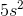. Then comes the d-block containing elements 39-48 that account for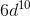. Finally comes the p-block containing elements 49-53 that account for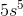. Therefore, the electron configuration of iodine in nobel gas configuration is